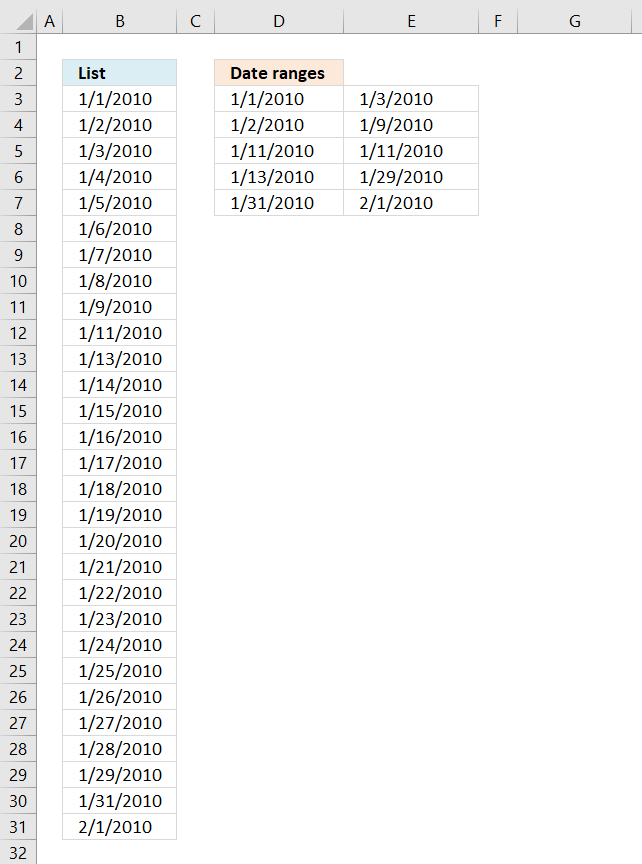Author: Oscar Cronquist Article last updated on December 23, 2018The array formula in cell B3 creates a list of dates based on the date ranges displayed in D3:E7, it handles overlapping date ranges, however, only one instance of each overlapping date is shown.

Array formula in cell B3:

=SMALL(IF(COUNTIFS(\$D\$3:\$D\$7, "<="&MIN(\$D\$3:\$E\$7)+ROW(\$B\$1:INDEX(\$B:\$B, MAX(\$D\$3:\$E\$7)-MIN(\$D\$3:\$E\$7)+1))-1, \$E\$3:\$E\$7, ">="&MIN(\$D\$3:\$E\$7)+ROW(\$B\$1:INDEX(\$B:\$B, MAX(\$D\$3:\$E\$7)-MIN(\$D\$3:\$E\$7)+1))-1), MIN(\$D\$3:\$E\$7)+ROW(\$B\$1:INDEX(\$B:\$B, MAX(\$D\$3:\$E\$7)-MIN(\$D\$3:\$E\$7)+1))-1, ""), ROWS(\$B\$1:B1))

How to create an array formula

1. Copy above array formula
2. Select cell B3
3. Press with left mouse button on in formula bar4. Paste array formula in formula bar
5. Press and hold Ctrl + Shift
6. Press Enter
7. Release all keys

How to copy array formula

1. Select cell B3
2. Copy cell (Ctrl + c)
3. Select cell range B3:B30
4. Paste (Ctrl + v)

### Explaining formula in cell B3

#### Step 1 - Create a dynamiccell reference

This step calculates the first date and the last date of all date ranges and builds a cell reference with as many rows as there are days between the first date and the last date.

The MIN function returns the smallest number (date) from all date ranges and the MAX function returns largest number (date) from all date ranges.

\$B\$1:INDEX(\$B:\$B, MAX(\$D\$3:\$E\$7)-MIN(\$D\$3:\$E\$7)+1)

becomes

\$B\$1:INDEX(\$B:\$B, 40210-40179+1)

becomes

\$B\$1:INDEX(\$B:\$B, 32)

and returns

\$B\$1:\$B\$32

#### Step 2 - Create a sequence of values

The ROW function creates a sequence of numbers between 0 (zero) and n based on the number of rows in the cell reference.

ROW(\$B\$1:INDEX(\$B:\$B, MAX(\$D\$3:\$E\$7)-MIN(\$D\$3:\$E\$7)+1))-1

becomes

ROW(\$B\$1:\$B\$32)-1

and returns

{0; 1; 2; 3; 4; 5; 6; 7; 8; 9; 10; 11; 12; 13; 14; 15; 16; 17; 18; 19; 20; 21; 22; 23; 24; 25; 26; 27; 28; 29; 30; 31}.

#### Step 3 - Create an array of dates

"<="&MIN(\$D\$3:\$E\$7)+ROW(\$B\$1:INDEX(\$B:\$B, MAX(\$D\$3:\$E\$7)-MIN(\$D\$3:\$E\$7)+1))-1

becomes

"<="&MIN(\$D\$3:\$E\$7)+{0; 1; 2; 3; 4; 5; 6; 7; 8; 9; 10; 11; 12; 13; 14; 15; 16; 17; 18; 19; 20; 21; 22; 23; 24; 25; 26; 27; 28; 29; 30; 31}

The MIN function returns the smallest number (earliest date).

"<="&MIN(\$D\$3:\$E\$7)+ROW(\$B\$1:INDEX(\$B:\$B, MAX(\$D\$3:\$E\$7)-MIN(\$D\$3:\$E\$7)+1))-1

becomes

"<="&MIN(\$D\$3:\$E\$7)+{0; 1; 2; 3; 4; 5; 6; 7; 8; 9; 10; 11; 12; 13; 14; 15; 16; 17; 18; 19; 20; 21; 22; 23; 24; 25; 26; 27; 28; 29; 30; 31}

becomes

"<="&40179+{0; 1; 2; 3; 4; 5; 6; 7; 8; 9; 10; 11; 12; 13; 14; 15; 16; 17; 18; 19; 20; 21; 22; 23; 24; 25; 26; 27; 28; 29; 30; 31}

becomes

"<="&{40179; 40180; 40181; 40182; 40183; 40184; 40185; 40186; 40187; 40188; 40189; 40190; 40191; 40192; 40193; 40194; 40195; 40196; 40197; 40198; 40199; 40200; 40201; 40202; 40203; 40204; 40205; 40206; 40207; 40208; 40209; 40210}

and returns

{"<=40179"; "<=40180"; "<=40181"; "<=40182"; "<=40183"; "<=40184"; "<=40185"; "<=40186"; "<=40187"; "<=40188"; "<=40189"; "<=40190"; "<=40191"; "<=40192"; "<=40193"; "<=40194"; "<=40195"; "<=40196"; "<=40197"; "<=40198"; "<=40199"; "<=40200"; "<=40201"; "<=40202"; "<=40203"; "<=40204"; "<=40205"; "<=40206"; "<=40207"; "<=40208"; "<=40209"; "<=40210"}

The less than sign and equal sign is there to check if dates are inside the date range, this is calculated in the next step.

#### Step 4 - Calcualte if date is in date range

The COUNTIFS function calculates the number of cells across multiple ranges that equals all given conditions.

COUNTIFS(\$D\$3:\$D\$7, "<="&MIN(\$D\$3:\$E\$7)+ROW(\$B\$1:INDEX(\$B:\$B, MAX(\$D\$3:\$E\$7)-MIN(\$D\$3:\$E\$7)+1))-1, \$E\$3:\$E\$7, ">="&MIN(\$D\$3:\$E\$7)+ROW(\$B\$1:INDEX(\$B:\$B, MAX(\$D\$3:\$E\$7)-MIN(\$D\$3:\$E\$7)+1))-1)

returns

{1; 2; 2; 1; 1; 1; 1; 1; 1; 0; 1; 0; 1; 1; 1; 1; 1; 1; 1; 1; 1; 1; 1; 1; 1; 1; 1; 1; 1; 0; 1; 1}

A 0 (zero) indicates that the date is not in a date range, 1 means that the date is in one date range, 2 means two dates ranges and so on.

#### Step 5 - Replace any number except 0 (zero) with corresponding date

The IF function has three arguments, the first one must be a logical expression. If the expression evaluates to TRUE then one thing happens (argument 2) and if FALSE another thing happens (argument 3).

IF(COUNTIFS(\$D\$3:\$D\$7, "<="&MIN(\$D\$3:\$E\$7)+ROW(\$B\$1:INDEX(\$B:\$B, MAX(\$D\$3:\$E\$7)-MIN(\$D\$3:\$E\$7)+1))-1, \$E\$3:\$E\$7, ">="&MIN(\$D\$3:\$E\$7)+ROW(\$B\$1:INDEX(\$B:\$B, MAX(\$D\$3:\$E\$7)-MIN(\$D\$3:\$E\$7)+1))-1), MIN(\$D\$3:\$E\$7)+ROW(\$B\$1:INDEX(\$B:\$B, MAX(\$D\$3:\$E\$7)-MIN(\$D\$3:\$E\$7)+1))-1, "")

becomes

IF({1; 2; 2; 1; 1; 1; 1; 1; 1; 0; 1; 0; 1; 1; 1; 1; 1; 1; 1; 1; 1; 1; 1; 1; 1; 1; 1; 1; 1; 0; 1; 1}, MIN(\$D\$3:\$E\$7)+ROW(\$B\$1:INDEX(\$B:\$B, MAX(\$D\$3:\$E\$7)-MIN(\$D\$3:\$E\$7)+1))-1, "")

becomes

IF({1; 2; 2; 1; 1; 1; 1; 1; 1; 0; 1; 0; 1; 1; 1; 1; 1; 1; 1; 1; 1; 1; 1; 1; 1; 1; 1; 1; 1; 0; 1; 1}, {40179; 40180; 40181; 40182; 40183; 40184; 40185; 40186; 40187; 40188; 40189; 40190; 40191; 40192; 40193; 40194; 40195; 40196; 40197; 40198; 40199; 40200; 40201; 40202; 40203; 40204; 40205; 40206; 40207; 40208; 40209; 40210}, "")

and returns

{40179; 40180; 40181; 40182; 40183; 40184; 40185; 40186; 40187; ""; 40189; ""; 40191; 40192; 40193; 40194; 40195; 40196; 40197; 40198; 40199; 40200; 40201; 40202; 40203; 40204; 40205; 40206; 40207; ""; 40209; 40210}.

#### Step 6 - Extract k-th smallest date

To be able to return a new value in a cell each I use the SMALL function to filter date numbers from smallest to largest.

SMALL(IF(COUNTIFS(\$D\$3:\$D\$7, "<="&MIN(\$D\$3:\$E\$7)+ROW(\$B\$1:INDEX(\$B:\$B, MAX(\$D\$3:\$E\$7)-MIN(\$D\$3:\$E\$7)+1))-1, \$E\$3:\$E\$7, ">="&MIN(\$D\$3:\$E\$7)+ROW(\$B\$1:INDEX(\$B:\$B, MAX(\$D\$3:\$E\$7)-MIN(\$D\$3:\$E\$7)+1))-1), MIN(\$D\$3:\$E\$7)+ROW(\$B\$1:INDEX(\$B:\$B, MAX(\$D\$3:\$E\$7)-MIN(\$D\$3:\$E\$7)+1))-1, ""), ROWS(\$B\$1:B1))

becomes

SMALL({40179; 40180; 40181; 40182; 40183; 40184; 40185; 40186; 40187; ""; 40189; ""; 40191; 40192; 40193; 40194; 40195; 40196; 40197; 40198; 40199; 40200; 40201; 40202; 40203; 40204; 40205; 40206; 40207; ""; 40209; 40210}, ROWS(\$B\$1:B1))

becomes

SMALL({40179; 40180; 40181; 40182; 40183; 40184; 40185; 40186; 40187; ""; 40189; ""; 40191; 40192; 40193; 40194; 40195; 40196; 40197; 40198; 40199; 40200; 40201; 40202; 40203; 40204; 40205; 40206; 40207; ""; 40209; 40210}, 1)

and returns 1/1/2010 in cell B3.

### Get the Excel fileCreate-dates-from-date-ranges2-1.xlsx